# Algebra II: Trigonometry Chapter Exam

Exam Instructions:

Choose your answers to the questions and click 'Next' to see the next set of questions. You can skip questions if you would like and come back to them later with the yellow "Go To First Skipped Question" button. When you have completed the practice exam, a green submit button will appear. Click it to see your results. Good luck!

### Page 1

#### Question 2 2. Solve the following trigonometric equation for θ.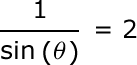### Page 2

#### Question 6 6. In the pictured triangle, ∠A is 62 degrees and ∠C is 86 degrees. If side a is 112 units long, approximately how long is side c?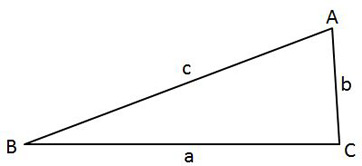#### Question 7 7. Simplify the following expression. Report the answer in degrees.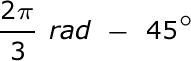#### Question 8 8. What is the function for the following graph?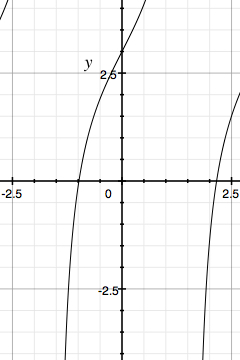#### Question 9 9. Convert the following measurement in radian to degrees.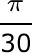### Page 3

#### Question 13 13. What's the value of 2sin(θ)cos(θ) given that the equation below is true?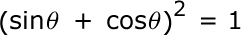### Page 4

#### Question 19 19. Solve the following equation for x.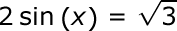#### Question 20 20. In the pictured triangle, ∠A is 137 degrees and ∠B is 28 degrees. If side b is 71 units long, approximately how long is side a?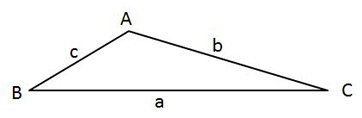### Page 5

#### Question 21 21. Simplify the following expression.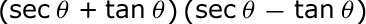#### Question 22 22. In the pictured triangle, ∠A is 58 degrees and ∠C is 63 degrees. If side a is 84 units long, approximately how long is side c?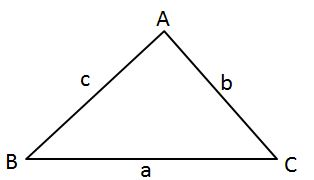#### Question 23 23. Solve for the value of x in the trigonometric equation below. (Use the domain -π/2 to π/2)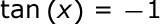#### Question 24 24. What is the value of cos(θ) given that the equation below is true?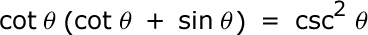### Page 6

#### Question 30 30. What's the value of sec2 (θ) given that the equation below is true?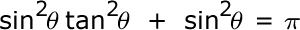#### Algebra II: Trigonometry Chapter Exam Instructions

Choose your answers to the questions and click 'Next' to see the next set of questions. You can skip questions if you would like and come back to them later with the yellow "Go To First Skipped Question" button. When you have completed the practice exam, a green submit button will appear. Click it to see your results. Good luck!

Support